# Electronics and Communication Engineering - Exam Questions Papers

21.

Total electric field intensity is given as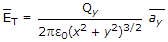(V/m). The maximum value of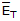will occur; if and only if

 A.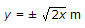B.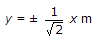C.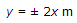D.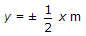Explanation:

To find value of y at which E will be maximum, differentiable ET with respect to y and set it equal to zero.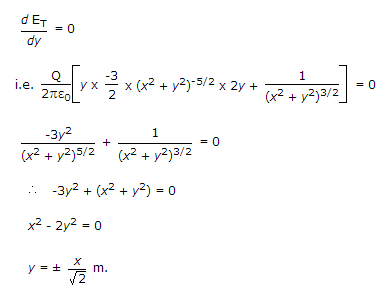22.

The effective length of an 1/2 antenna (Given Rr = 73 Ω and (Ae)max = 0.34λ2 and η = 120p) is __________ .

 A. 0.6348 λ B. 0.5133 λ C. 0.1587 λ D. 0.3714 λ

Explanation: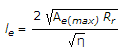=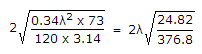le = 0.5133λ.

23.

Find Z11,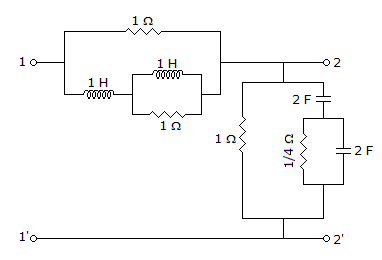A. 2 Ω B. 4 Ω C. 1 Ω D.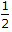Ω

Explanation: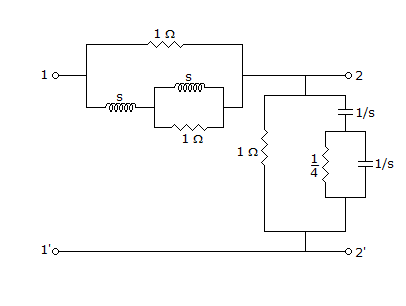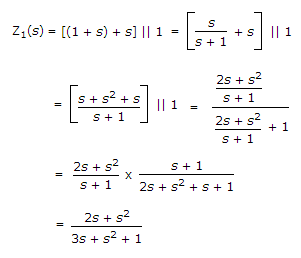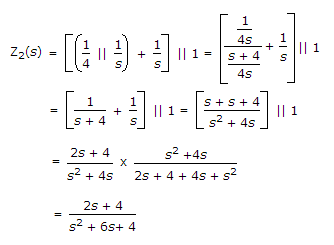Z11(s) = Z1(s) + Z2(s) = 1 Ω.

24.

Consider a Binary Symmetric Channel (BSC) with probability of error being p. To transmit a bit, say 1, we transmit a sequence of three 1 s. The receiver will interpret the received sequence to represent 1 if at least two bits are 1. The probability that the transmitted bit will be received in error is

 A. p3 + 3p2 (1 - p) B. p3 C. (1 - p)3 D. p3 + p2 (1 -p)

Explanation:

Probability of error = P

Thus probability of no error = (1 - P)

Now probability that transmitted bit, received in error

= all bits are with error + one bit is with error

= P3 + 3C1P2 (1 - P) = P3 + 3P2 (1 - P).

25.

Consider the sequence x(n) whose fourier transform X(e) is depicted for - p ≤ ω ≤ p as shown in figure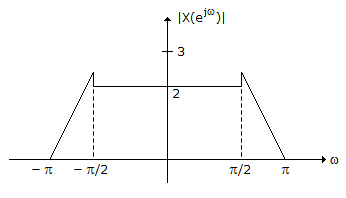1. x(n) is periodic
2. Real
3. Even
4. Finite energy

 A. 1 - True, 2 - True, 3 - True, 4 - True B. 1 - False, 2 - True, 3 - False, 4 - True C. 1 - True, 2 - False, 3 - True, 4 - False D. 1 - False, 2 - False, 3 - False, 4 - True

Explanation:

Accordingly, we note first that periodicity in the time domain implies that the Fourier transform is zero, except possibly for impulses located at various integer multiples of the fundamental frequency.

This is not true for X(e). We conclude, then, that x[n] is not periodic.

Next, from the symmetry properties for Fourier Transforms, we know that a real valued sequence must have a Fourier transform of even magnitude and a phase function that is odd.

This is true for the given |X(e)| and ∠X(e). We thus conclude that x[n] is real.

Third, if x[n] is an even function, then, by the symmetry properties for real signals, X(e) must be real and even.

However, since X(e) = |X(e)| e-j2ω, X(e) is not a real-valued function.

Consequently, x[n] is not even.

Finally, to test for the finite-energy property, we may use Parseval's relation,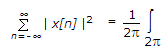|X(e)|2

It is clear that integrating |X(e)|2 from - p to p will yield a finite quantity.

We conclude that x[n] has finite energy.

#### Current Affairs 2022

Interview Questions and Answers